#### 期刊菜单

Analysis of Influence of Closing Process of Ball Valve on Water Hammer Effect
DOI: 10.12677/IJM.2021.104023, PDF, HTML, XML, 下载: 167  浏览: 607

Abstract: In this paper, in order to analyze the influence of water hammer effect in different closing processes of ball valve, the sliding grid of fluent software and udf technology are used to simulate the rotation process of ball valve when it is closed, and the changes of flow field and water hammer pressure during the closing process of ball valve are studied and analyzed. The results show that with the closing of the valve, the flow rate of the ball valve increases first and then decreases. When the flow rate of the ball valve reaches the maximum value, the fluid medium has the maximum impact on the valve core, and the water hammer pressure of the ball valve reaches the maximum value. The maximum water hammer pressure of ball valve gradually decreases with the increase of valve closing time, and under the same valve closing time, uniform acceleration and uniform deceleration will produce greater water hammer pressure than uniform speed.

1. 引言

2. 流动控制方程 

$\frac{\partial \rho }{\partial t}+\frac{\rho \partial {u}_{i}}{\partial {x}_{i}}=0$ (1)

$\frac{\partial }{\partial t}\left(\rho {u}_{i}\right)+\frac{\partial }{\partial {x}_{i}}\left(\rho {u}_{j}{u}_{i}\right)=-\frac{\partial p}{\partial {x}_{i}}+\frac{\partial }{\partial {x}_{i}}\left[\left(\mu +{\mu }_{1}\right)\left(\frac{\partial {u}_{i}}{\partial {x}_{i}}+\frac{\partial {u}_{j}}{\partial {x}_{i}}\right)\right]$ (2)

k (湍动能)方程：

$\frac{\partial \left(\rho k\right)}{\partial t}+\frac{\partial \left(\rho k{\mu }_{i}\right)}{\partial {x}_{i}}=\frac{\partial }{\partial {x}_{i}}\left[\left(\mu +\frac{{\mu }_{t}}{{\sigma }_{k}}\right)\frac{\partial k}{\partial {x}_{j}}\right]+{G}_{k}+{G}_{b}-{\rho }_{\in }+{Y}_{M}+{S}_{k}$ (3)

$\epsilon$ (湍流耗散率)方程：

$\frac{\partial \left(\rho \epsilon \right)}{\partial t}+\frac{\partial \left(\rho \epsilon {\mu }_{i}\right)}{\partial {x}_{i}}=\frac{\partial }{\partial {x}_{i}}\left[\left(\mu +\frac{{\mu }_{t}}{{\sigma }_{\epsilon }}\right)\frac{\partial \epsilon }{\partial {x}_{j}}\right]+{C}_{1\epsilon }\frac{\epsilon }{k}\left({G}_{k}+{C}_{3\epsilon }{G}_{b}\right)-{C}_{2\epsilon }\rho \frac{{\epsilon }^{2}}{k}+{S}_{\epsilon }$ (4)

3. 数值模拟计算

3.1. 几何模型及其网格划分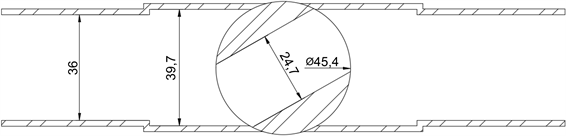Figure 1. Internal structure diagram of ball valve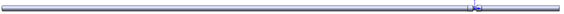Figure 2. Geometric model drawing

3.2. 边界条件

3.3. 计算结果分析

3.3.1. 球阀不同开度下流场分析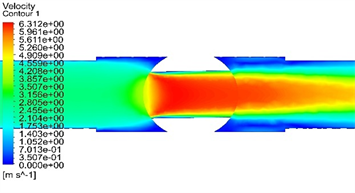(a) 阀门开度为90˚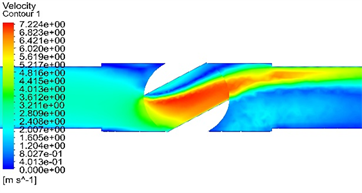(b) 阀门开度为60˚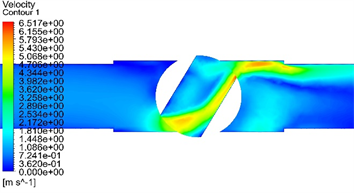(c) 阀门开度为30˚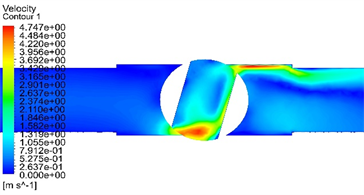(d) 阀门开度为15˚

Figure 3. Ball valve velocity nephogram

3.3.2. 球阀关阀时间对水锤压力的影响Figure 4. Water hammer pressure at different valve closure times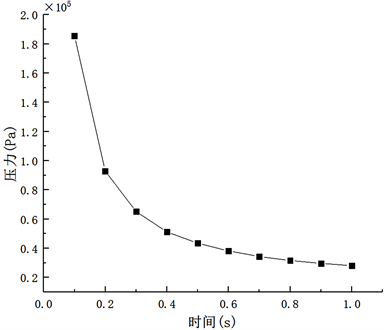Figure 5. Maximum water hammer pressure variation curveTable 1. Maximum water hammer pressure value and reduction rate

3.3.3. 球阀关阀规律对水锤压力的影响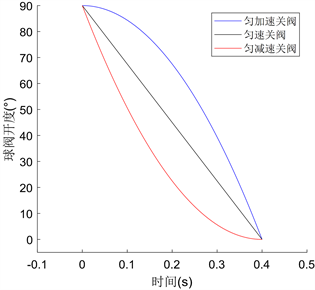Figure 6. Valve opening change curve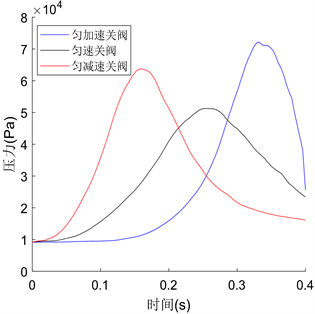Figure 7. Water hammer pressure at different valve closure times

4. 结论

1) 在球阀所有开度下，阀芯开口处产生最大速度，通过开口后，压强开始下降，速度也开始减小。在球阀关阀过程中，随着阀门不断关闭，流场逐渐变得复杂，阀芯开口处逐渐出现涡流，球阀流速先增大后减小，最大流速出现在30˚~60˚之间。

2) 球阀关阀过程中水锤压力会随着关阀时间的增加不断减小，当球阀关阀时间减小到一定数值后再增加关阀时间对水锤压力的影响不再明显，故适当提高关阀时间可以很好地抑制水锤效应的影响。

3) 球阀在同样关阀时间下，采用匀速关阀相较于匀加速、匀减速关阀有着更小水锤压力，且匀速关阀压力变化过程更为平缓，故采用匀速关阀能更好地起到水锤防护的作用。

  刘竹溪, 刘光临. 泵站水锤及其防护[M]. 北京: 水利电力出版社, 1988.  彭利坤, 张阳阳, 邢继峰. 舰船纵倾均衡水舱加气调水时管路冲击特性研究[J]. 液压与气动, 2013(8): 9-12.  潘子超, 阮欣, 陈艾荣. 基于任意级配的二维随机骨料生成方法[J]. 同济大学学报(自然科学版), 2013, 41(5): 759-764.  Bazant, Z.P. and Tab-bara, M.R. (1990) Random Particle Models for Fracture of Aggregate or Fiber Composites. Journal of Engineering Mechanics, 116, 1686-1705. https://doi.org/10.1061/(ASCE)0733-9399(1990)116:8(1686)  王学芳, 叶宏开, 汤荣铭. 工业管道中的水锤[M]. 北京: 科学出版社, 1995.  华晔, 廖伟丽. CFD技术在管道阀门水击计算中的应用[J]. 电网与清洁能源, 2009, 25(3): 72-75.  马康, 顾振杰, 白长青. 改进的特征线方法及过载对水锤效应影响分析[J]. 计算力学学报, 2020, 37(5): 623-628.  刘华坪, 陈浮, 马波. 基于动网格与UDF技术的阀门流场数值模拟[J]. 汽轮机技术, 2008(2): 106-108.  郭兰兰, 耿介, 石硕, 苑飞, 雷丽, 杜广生. 基于UDF方法的阀门变速关闭过程中的水击压强计算研究[J]. 山东大学学报(理学版), 2014, 49(3): 27-30.  许文奇, 彭利坤, 屈铎. 基于CFD的球阀关闭过程水击现象研究[J]. 机床与液压, 2017, 45(11): 170-174.  韩占忠, 王敬, 兰小平. FLUENT流体工程仿真计算实例与应用[M]. 北京: 北京理工大学出版社, 2004.